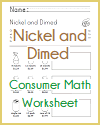Grade 4 Math: Operations and Algebraic Thinking | Student Handouts

Grade 4 Math: Operations and Algebraic Thinking
 www.studenthandouts.com > Grade 4 > Grade 4 Math > Grade 4 Operations & Algebraic Thinking
Use the four operations with whole numbers to solve problems.
CCSS.MATH.CONTENT.4.OA.A.1: Interpret a multiplication equation as a comparison, e.g., interpret 35 = 5 × 7 as a statement that 35 is 5 times as many as 7 and 7 times as many as 5. Represent verbal statements of multiplicative comparisons as multiplication equations.
CCSS.MATH.CONTENT.4.OA.A.2: Multiply or divide to solve word problems involving multiplicative comparison, e.g., by using drawings and equations with a symbol for the unknown number to represent the problem, distinguishing multiplicative comparison from additive comparison.Consumer Math Worksheet
CCSS.MATH.CONTENT.4.OA.A.3: Solve multistep word problems posed with whole numbers and having whole-number answers using the four operations, including problems in which remainders must be interpreted. Represent these problems using equations with a letter standing for the unknown quantity. Assess the reasonableness of answers using mental computation and estimation strategies including rounding.
Gain familiarity with factors and multiples.
CCSS.MATH.CONTENT.4.OA.B.4: Find all factor pairs for a whole number in the range 1-100. Recognize that a whole number is a multiple of each of its factors. Determine whether a given whole number in the range 1-100 is a multiple of a given one-digit number. Determine whether a given whole number in the range 1-100 is prime or composite.
Generate and analyze patterns.
CCSS.MATH.CONTENT.4.OA.C.5: Generate a number or shape pattern that follows a given rule. Identify apparent features of the pattern that were not explicit in the rule itself. For example, given the rule "Add 3" and the starting number 1, generate terms in the resulting sequence and observe that the terms appear to alternate between odd and even numbers. Explain informally why the numbers will continue to alternate in this way.